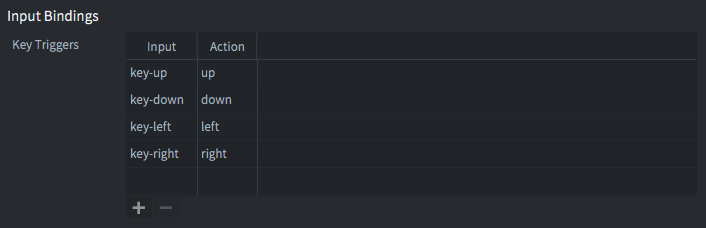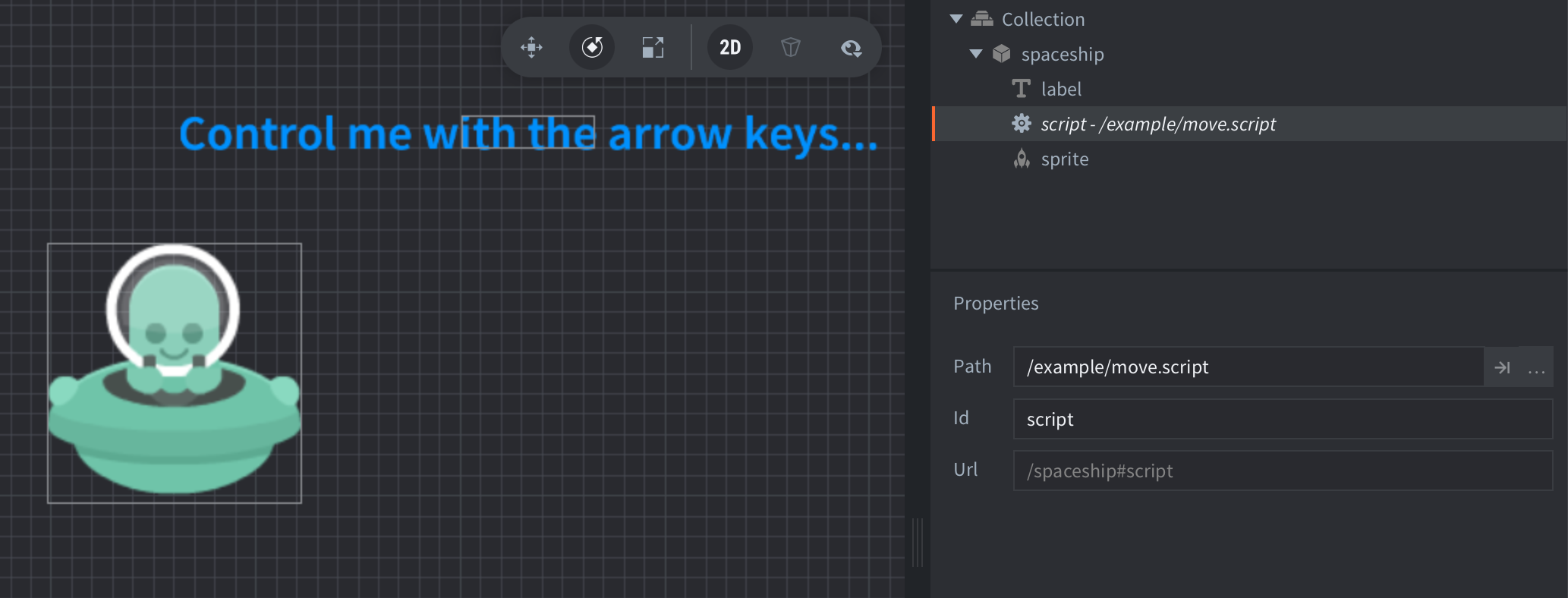#### Setup#### Scripts

move.script

```function init(self)
msg.post(".", "acquire_input_focus") -- <1>
self.vel = vmath.vector3() -- <2>
end

function update(self, dt)
local pos = go.get_position() -- <3>
pos = pos + self.vel * dt -- <4>
go.set_position(pos) -- <5>

self.vel.x = 0 -- <6>
self.vel.y = 0
end

function on_input(self, action_id, action)
if action_id == hash("up") then
self.vel.y = 150 -- <7>
elseif action_id == hash("down") then
self.vel.y = -150
elseif action_id == hash("left") then
self.vel.x = -150 -- <8>
elseif action_id == hash("right") then
self.vel.x = 150
end
end

--[[
1. Tell the engine that the current game object ("." is
shorthand for that) should receive user input to the function
`on_input()` in its script components.
2. Construct a vector to indicate velocity. It will initially be
zero.
3. Each frame, get the current position and store in `pos`.
4. Add the velocity, scaled to the current frame length. Velocity
is therefore expressed in pixels per second.
5. Set the game object's position to the newly calculated position.
6. Zero out the velocity. If no input is given, there should be
no movement.
7. If the user presses "up", set the y component of the velocity to 150.
If the user presses "down", set the y component to -150.
8. Similarly, if the user presses "left", set the x component of the velocity to -150.
And finally, if the user presses "right", set the x component to 150.
--]]```

If you want to play with these examples, you can get the project on Github.

Do you want to see more examples? Why not write a few yourself and submit a pull request? We love contributions.